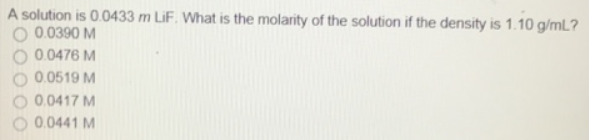# Problem: A solution is 0.0433 m LiF. What is the molarity of the solution if the density is 1.10 g/mL? (i) 0.0390 M (ii) 0.0476 M (iii) 0.0519 M (iv) 0.0417M (v) 0.0441 M

###### FREE Expert Solution
80% (264 ratings)###### Problem Details

A solution is 0.0433 m LiF. What is the molarity of the solution if the density is 1.10 g/mL?

(i) 0.0390 M

(ii) 0.0476 M

(iii) 0.0519 M

(iv) 0.0417M

(v) 0.0441 M